# RFEM

Online manuals, introductory examples, tutorials, and other documentation.

# 6.2 Member Loads

General description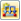Member loads are forces, moments, temperature actions, or imposed deformations that act on members.

To apply a member load, a member must already be defined.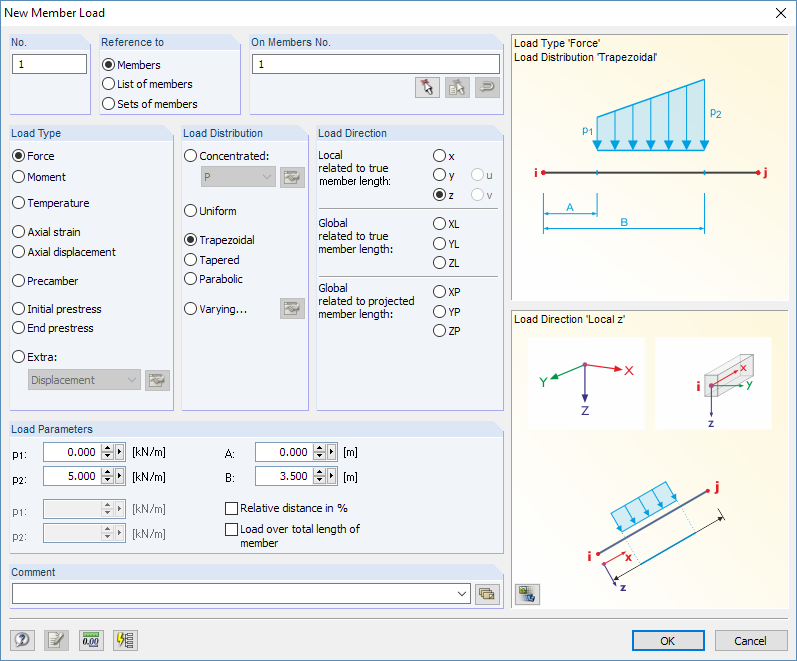Image 6.15 New Member Load dialog box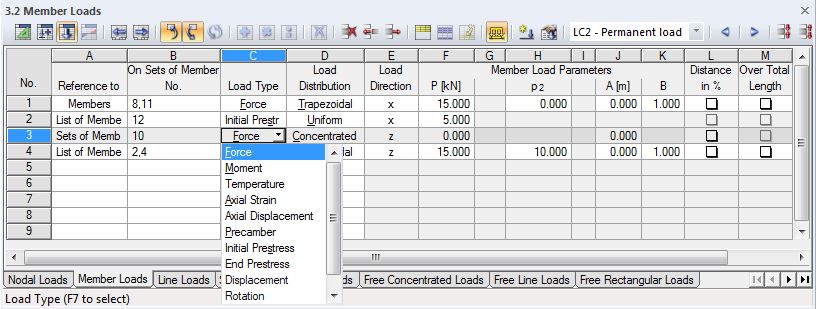Image 6.16 Table 3.2 Member Loads

The member load's number is automatically assigned in the New Member Load dialog box, but it can also be changed there. The numerical order is not important.

Reference to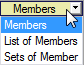Define the model elements to which you want to apply the member load. The following options can be selected:

Members

The load acts on one member or on each member among several.

List of members

The load acts on all members, which have to be specified in a list. Thus, when trapezoidal member loads are used, load parameters are not applied individually to each member but as a total load to all members of the member list. The load effects of a trapezoidal member load on single members in contrast to a member list are shown in Figure 6.17.

You can use a member list to apply loads over all members without defining continuous members. Moreover, it is possible to quickly change the load reference to individual members.

Sets of members

The load acts on a set of members or on each among several sets of members. Similar to the member list described above, load parameters are applied to all of the members included in the member set.

Sets of members are divided into continuous members and groups of members (see Chapter 4.21). While it is no problem to apply loads on sets of members to continuous members, member groups have to be handled with care: The reference to a member group is usually problematic for trapezoidal loads.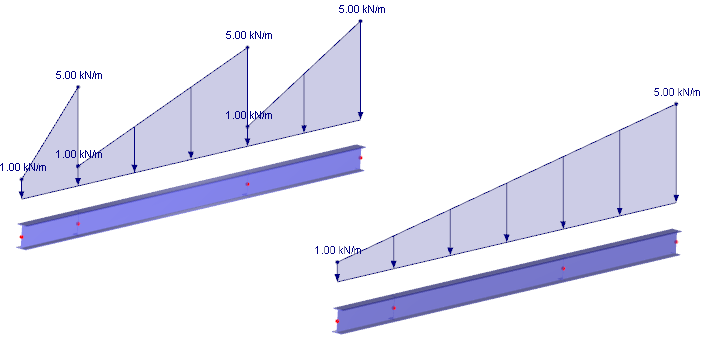Image 6.17 Trapezoidal load with reference to members (left) and to a list of members (right)
On Members No.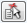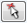In the text box, enter the numbers of the members or sets of members on which the load acts. You can also select nodes graphically in the dialog box.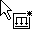If you have selected graphical input by clicking the toolbar button, you have to enter the load data first. After clicking [OK], you can select the relevant members or sets of members one by one in the work window.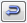For trapezoidal or variable loads with load reference to a member list, you can adjust the member numbers by using the [Reverse Orientation of Members] dialog button shown on the left.

Load Type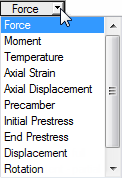Specify the load type in this dialog section. Depending on your selection, certain parts of the dialog box and columns of the table are disabled. The following load types can be selected:

Table 6.1 Load types

Force

Concentrated load, distributed load, or trapezoidal load
The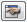button allows you to set a load eccentricity.

Moment

Concentrated moment, distributed moment, or trapezoidal moment

Temperature

Temperature load uniformly distributed over member cross-section, or temperature difference between top and bottom side of member
Load applied as uniform or trapezoidal over member length or as trapezoidal over cross-section. A positive load value means that the member or the upper side is heating.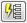Axial strain

Imposed tensile or compressive strain ε of member
A positive load value means that the member is extended. Thus, a prestress as a member contraction must be entered negatively.
Use the dialog button shown on the left to determine the strain due to shrinkage from the parameters for contraction and drying shrinkage (see Figure 6.25).

Axial displacement

Imposed tensile or compressive strain Δl of member

Precamber

Imposed curvature of member

Initial prestress

Prestressing force that acts on the member before calculation
A positive load value means that the member is extended.

End prestress

Axial force expected to be available on member after calculation (not possible for rigid members and cables)
A positive load value means that the member is extended.

Displacement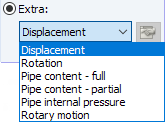Displacement by quantity Δ for determination of influence lines

Rotation

Rotation about angle φ for influence lines

Pipe content - full

Distributed load due to complete filling of pipe
Specify weight density γ of pipe content.

Pipe content - partial

Distributed load due to partial filling of pipe
In addition to weight density γ of pipe content, specify filling height d.

Pipe internal pressure

Constant internal pressure of a pipe

Rotary motion

Centrifugal force from mass and angular velocity ω on member
The rotation axis can be defined by using thebutton.

The graphic in the upper right of the dialog box shows the selected load type including the influence of the signs of forces and strains.

Load Distribution

The Load Distribution dialog section provides different options for displaying the effect of the load. The dialog graphic in the top right corner may be helpful.

Table 6.2 Load distributions
Load Distribution Diagram Description

Concentrated P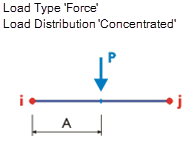Concentrated load, concentrated moment
Specify the size of the concentrated load or concentrated moment and the distance of the point of load application in relation to the member start as Member Load Parameters.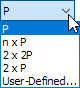Multiple loads

Concentrated n x P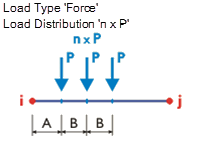Multiple concentrated loads or moments
The list provides several arrangement options for load pairs or multiple concentrated loads such as axle loads. You can also define User-defined loads in a separate dialog box, which can be opened with thebutton.
The option shown on the left is suitable for single forces, which are equal in size and act at a uniform distance.
Define the size of the concentrated load, the distance between first load and member start, and the spacing between the load as Member Load Parameters.

Uniform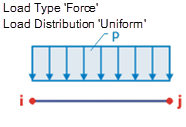Uniformly distributed load, uniformly distributed moment
Specify the size of the uniform member load or uniform moment as Member Load Parameters.

Trapezoidal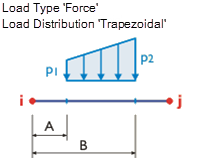Trapezoidal load, trapezoidal moment
As Member Load Parameters, define both load values and distances for a linearly variable load distribution as shown in the dialog graphic. A triangular load is created by setting one load value to zero.
If the Relative distance in % check box is selected, the distances can be specified relative to the member length.

Tapered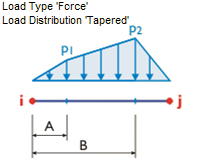Triangular-trapezoidal load, triangular-trapezoidal moment
For a linearly variable load distribution, specify the load values and distances as Load Parameters as shown in the dialog graphic.

Parabolic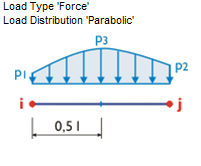Parabolic load, parabolic moment
The load acts as a parabolic load on the entire member. The load values at the member start and member end, as well as in the member center must be entered as Load Parameters.

Varying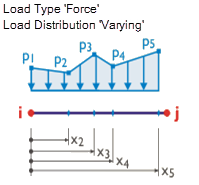Polygonally distributed load
Clicking thebutton opens the dialog box shown in Figure 6.18 where you can enter or import the parameters of the load distribution.

If you want to display a variable load, you can freely define the x-locations on the member with the corresponding load ordinates p. You only have to make sure that the x-locations are defined in an ascending order. Use the interactive graphic to check your input immediately.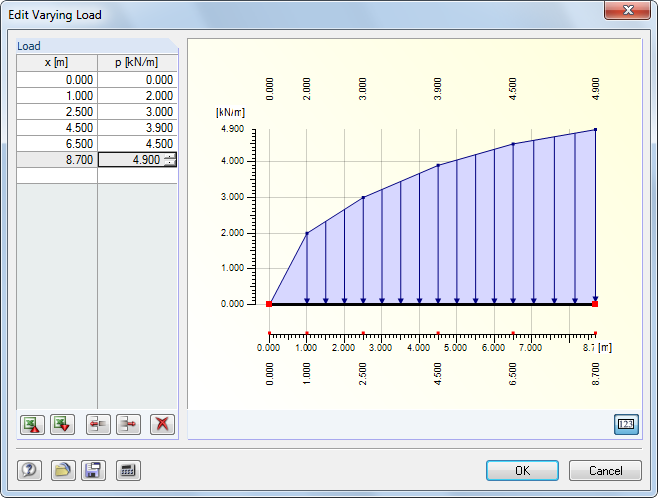Image 6.18 Edit Varying Load dialog box

The buttons in this dialog box have the following functions:

Table 6.3 Buttons in the Edit Varying Load dialog box
Button Function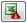Table export to MS Excel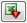Table import from MS Excel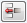Inserts a blank line above pointer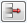Deletes active row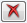Deletes all entries

Load Direction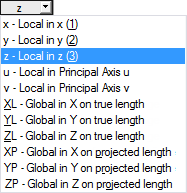The load can be effective in the direction of the global axes X, Y, Z, or the local member axes x, y, z or u, v (see Chapter 4.13). For a calculation according to the linear static analysis, it does not matter whether a load is defined as local or equivalent global. For geometrically nonlinear calculations, however, differences between locally and globally defined loads are possible: If the load is defined with a global direction of action, it keeps this direction when the finite elements start to twist. In case of a local direction of action, however, the load twists on the member according to the distortion of elements.

If the model type has been reduced to a planar system in the general data, you cannot access all load directions.

Local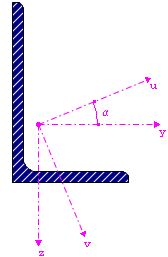The orientation of member axes is described in Chapter 4.17, paragraph Member Rotation. The local axis x represents the longitudinal axis of the member. For symmetrical sections, axis y represents the so-called 'strong' axis of the member cross-section, axis z accordingly the 'weak' axis. In case of asymmetrical cross-sections, loads can be related to the principal axes u and v, as well as the standard input axes y and z.

Examples for loads defined as local are temperature loads, wind loads that act on roof structures, or prestresses.

Global

The position of the local member axes is irrelevant for the load input if the load acts in direction of an axis of the global coordinate system XYZ.

Examples for loads defined as global are snow loads acting on roof constructions and wind loads on wall and gable columns.

The load impact can be related to different reference lengths:

• Related to true member length
• The load is applied to the entire member length.
• Related to projected member length in X / Y / Z
• The application length of the load is converted to the projection of the member in one of the directions of the global coordinate system. Select this option to, for example, define a snow load on the projected ground-plan area of a roof.

### Note

RFEM always applies member loads in the shear center. An intended torsion originating from the cross-sectional geometry (centroid unequal shear center) is not considered. Therefore, when asymmetrical cross-sections are used, a torsion moment determined from load multiplied by distance to the shear center must additionally be applied if, for example, loading is introduced in the centroid.

Member Load Parameters

In this dialog section / these table columns, the load values and, potentially, additional parameters are managed. The text boxes are labeled and accessible depending on the previously activated selection fields.

Load p1 / p2

Enter load values into the fields. Match the algebraic signs with the global or local orientations of axes. For prestresses, temperature changes, and axial strains, a positive value means that the member is strained and consequently extended.

When a trapezoidal load is selected, specify two load values. The dialog graphic in the upper right corner illustrates the load parameters.

Distance A / B

For concentrated and trapezoidal loads, enter the distances from the member start into these fields. The distances can also be defined relative to the member length by selecting the Relative distance in % check box (see below).

The dialog graphic in the upper right corner and the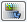button in the graphic below are useful when entering parameters.

### Note

If the Load Direction is projected to the member length XP, YP, or ZP, you also have to define the distances A and B in relation to the projected member length.

Relative distance in %

Select this check box to define the distances for concentrated or trapezoidal loads relative to the member length. Otherwise, the entries in the Distance text boxes described above represent absolute ranges.

Over Total Length

The check box can only be activated for trapezoidal loads. Select this option to arrange the linearly variable load from the member start to the member end. The Load Parameters A / B text boxes are no longer relevant and therefore disabled.

Example

In the following example, member loads are applied to a planar frame structure. You can see that it is not necessary to divide members by intermediate nodes to apply concentrated loads.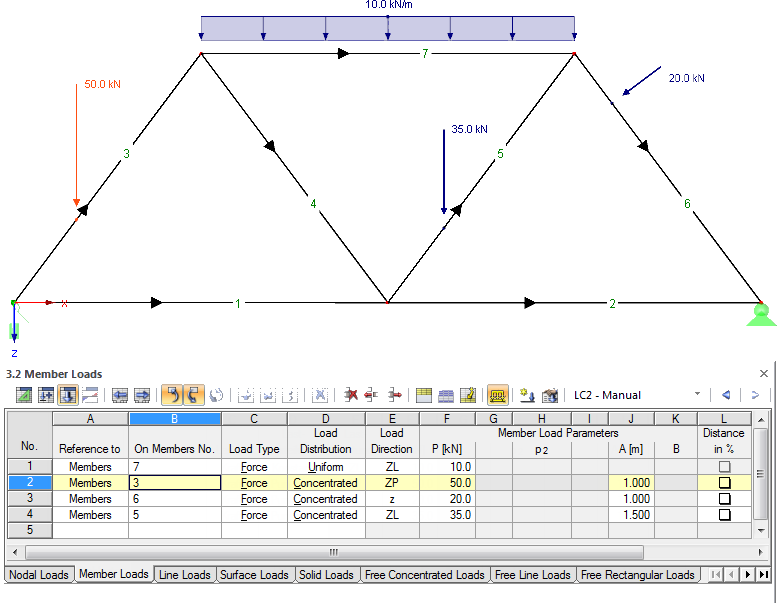Image 6.19 Framework with uniform load on upper chord and concentrated loads on diagonals